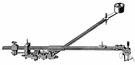integrator

(redirected from Active integrator circuit)
Also found in: Thesaurus, Medical, Encyclopedia.

in·te·gra·tor

(ĭn′tĭ-grā′tər)
n.
1. One that integrates.
2. An instrument for mechanically calculating definite integrals.

integrator

(ˈɪntɪˌɡreɪtə)
n
1. (Mathematics) a person or thing that integrates, esp a mechanical instrument that determines the value of a definite integral, as the area under a curve. See also planimeter
2. (Mathematics) computing
a. an arithmetic component with two input variables, x and y, whose output variable z is proportional to the integral of y with respect to x
b. an arithmetic component whose output variable is proportional to the integral of the input variable with respect to elapsed time

in•te•gra•tor

(ˈɪn tɪˌgreɪ tər)

n.
1. a person or thing that integrates.
2. an instrument for performing numerical integrations.
[1875–80]
ThesaurusAntonymsRelated WordsSynonymsLegend:
 Noun 1integrator - a measuring instrument for measuring the area of an irregular plane figureplanimetermeasuring device, measuring instrument, measuring system - instrument that shows the extent or amount or quantity or degree of something
Translations
intégrateurtransporteur intégral

integrator

[ˈɪntɪgreɪtəʳ] N
Site: Follow: Share:
Open / Close